# Digit sum

Determine for how many integers greater than 900 and less than 1,001 has digit sum digit of the digit sum number 1.

n =  1

### Step-by-step explanation: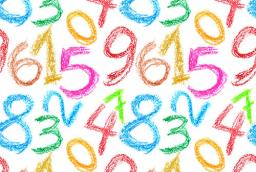Did you find an error or inaccuracy? Feel free to write us. Thank you!## Related math problems and questions:

• How manyHow many integers are greater than 547/3 and less than 931/4?
• How manyHow many numbers are less than 222 with a digit sum is 8?
• Two-digit numberI am a two-digit number less than 20. When I divide by three, then you get the rest one, and when you divide me by four, you get also rest 1. What number am I?
• Digit sumFind the smallest natural number n, the digit sum of which is equal to 37.
• Three-digit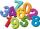How many three-digit natural numbers are greater than 321 if no digit in number repeated?
• How many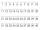How many double-digit numbers greater than 30 we can create from digits 0, 1, 2, 3, 4, 5? Numbers cannot be repeated in a two-digit number.
• PIN codePIN on Michael credit card is a four-digit number. Michael told this to his friend: • It is a prime number - that is, a number greater than 1, which is only divisible by number one and by itself. • The first digit is larger than the second. • The second d
• TissuesThe store got three kinds of tissues - 132 children, 156 women and 204 men. Tissues each species were packed into boxes after the number of pieces the same for all three types (and greatest). Determine the number, if you know that every box has more than
• Reminder and quotientThere are given numbers A = 135, B = 315. Find the smallest natural number R greater than 1 so that the proportions R:A, R:B are with the remainder 1.
• DigitsHow many natural numbers greater than 4000 which are formed from the numbers 0,1,3,7,9 with the figures not repeated, B) How many will the number of natural numbers less than 4000 and the numbers can be repeated?
• All pairs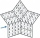Determine all pairs (m, n) of natural numbers for which is true: m s (n) = n s (m) = 70, where s (a) denotes the digit sum of the natural number a.
• Sum of sevenThe sum of seven consecutive odd natural numbers is 119. Determine the smallest of them.
• Digit sum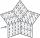How many are three-digit numbers that have a digit sum of 6?
• 7 digit numberIf 3c54d10 is divisible by 330, what is the sum of c and d?
• Unknown number 716% of the unknown number is by 21 less than unknown number itself. Determine the natural unknown number.
• Expressions with variableThis is algebra. Let n represent an unknown number and write the following expressions: 1. 4 times the sum of 7 and the number x 2. 4 times 7 plus the number x 3. 7 less than the product of 4 and the number x 4. 7 times the quantity 4 more than the number
• DivisibilityWrite all the integers x divisible by seven and eight simultaneously, for which the following applies: 100 < x < 200.